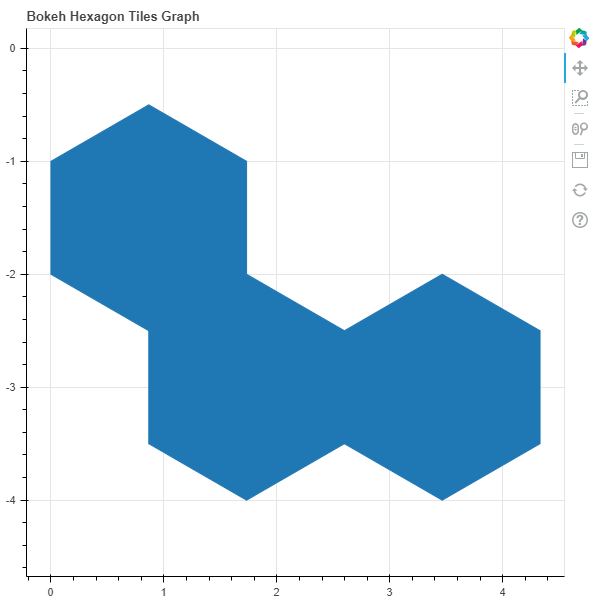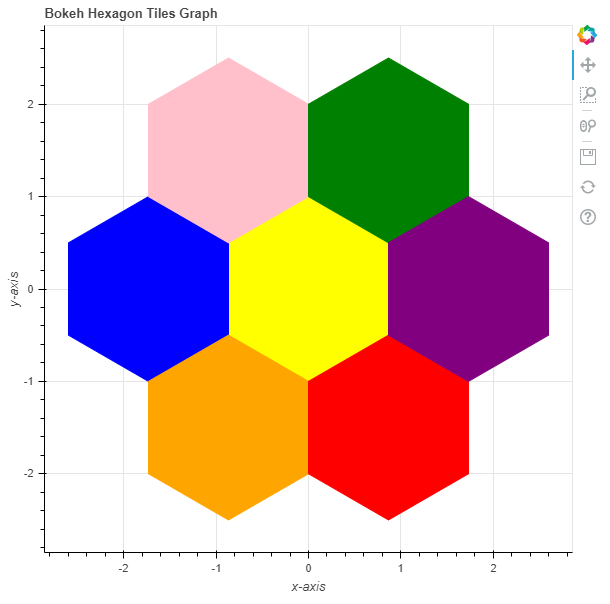# Python Bokeh – Plotting Hexagon Tiles on a Graph

• Last Updated : 10 Jul, 2020

Bokeh is a Python interactive data visualization. It renders its plots using HTML and JavaScript. It targets modern web browsers for presentation providing elegant, concise construction of novel graphics with high-performance interactivity.

Bokeh can be used to plot hexagon tiles on a graph. Plotting hexagon tiles on a graph can be done using the `hex_tile()` method of the `plotting` module.

## plotting.figure.hex_tile()

Syntax : hex_tile(parameters)

Parameters :

• q : column axial coordinates of the center of the hexagon tiles
• r : row axial coordinates of the center of the hexagon tiles
• aspect_scale : aspect scale value, default is 1
• fill_alpha : fill alpha value of the hexagon tile markers
• fill_color : fill color value of the hexagon tile markers
• line_alpha : percentage value of line alpha, default is 1
• line_cap : value of line cap for the line, default is butt
• line_color : color of the line, default is black
• line_dash : value of line dash such as : solid, dashed, dotted, dotdash, dashdot [default is solid ]
• line_dash_offset : value of line dash offset, default is 0
• line_join : value of line join, default in bevel
• line_width : value of the width of the line, default is 1
• name : user-supplied name for the model
• orientation : orientation value, default is pointytop
• scale : scale factor of individual tiles, default is 1
• size : radius of the hexagonal tiles, default is 1
• tags : user-supplied values for the model

Other Parameters :

• alpha : sets all alpha keyword arguments at once
• color : sets all color keyword arguments at once
• legend_field : name of a column in the data source that should be used
• legend_group : name of a column in the data source that should be used
• legend_label : labels the legend entry
• muted : determines whether the glyph should be rendered as muted or not, default is False
• name : optional user-supplied name to attach to the renderer
• source : user-supplied data source
• view : view for filtering the data source
• visible : determines whether the glyph should be rendered or not, default is True
• x_range_name : name of an extra range to use for mapping x-coordinates
• y_range_name : name of an extra range to use for mapping y-coordinates
• level : specifies the render level order for this glyph

Returns : an object of class `GlyphRenderer`

Example 1 : In this example we will be using the default values for plotting the graph. We have provided the size and fill_color attributes to make the glyph visible.

 `# importing the modules ``from` `bokeh.plotting ``import` `figure, output_file, show ``      ` `# file to save the model ``output_file(``"gfg.html"``) ``      ` `# instantiating the figure object ``graph ``=` `figure(title ``=` `"Bokeh Hexagon Tiles Graph"``,``               ``match_aspect ``=` `True``) ``    ` `# the points to be plotted ``r ``=` `[``0``, ``0``, ``1``] ``q ``=` `[``1``, ``2``, ``2``] ``   ` `# plotting the graph ``graph.hex_tile(r, q) ``    ` `# displaying the model ``show(graph) `

Output :Example 2 : In this example we will be plotting the hexagon tiles with various parameters

 `# importing the modules ``from` `bokeh.plotting ``import` `figure, output_file, show ``      ` `# file to save the model ``output_file(``"gfg.html"``) ``      ` `# instantiating the figure object ``graph ``=` `figure(title ``=` `"Bokeh Hexagon Tiles Graph"``,``               ``match_aspect ``=` `True``) `` ` `# name of the x-axis ``graph.xaxis.axis_label ``=` `"x-axis"``      ` `# name of the y-axis ``graph.yaxis.axis_label ``=` `"y-axis"``    ` `# the points to be plotted ``r ``=` `[``0``, ``-``1``,  ``0``,  ``1``, ``-``1``, ``0``, ``1``]``q ``=` `[``0``,  ``0``, ``-``1``, ``-``1``,  ``1``, ``1``, ``0``] `` ` `# fill color values``fill_color ``=` `[``"yellow"``, ``"blue"``, ``"pink"``, ``"green"``, ``"orange"``, ``"red"``, ``"purple"``]`` ` `# line color values``line_color ``=` `[``"yellow"``, ``"blue"``, ``"pink"``, ``"green"``, ``"orange"``, ``"red"``, ``"purple"``]`` ` `# plotting the graph ``graph.hex_tile(r, q,``               ``fill_color ``=` `fill_color,``               ``line_color ``=` `line_color) ``    ` `# displaying the model ``show(graph) `

Output :My Personal Notes arrow_drop_up